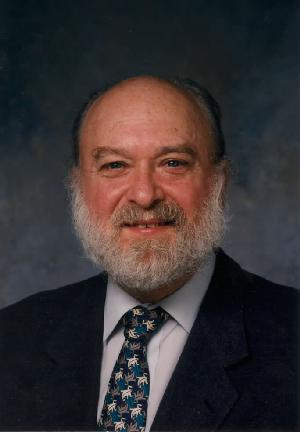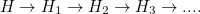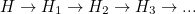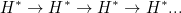# Going with the flow — part II

Marianne Freiberger and Rachel Thomas

In the first part of this article we saw how statistical physics provided a way of zooming in and out of a system to examine it on many scales. Kadanoff's block spin method is an example of a powerful general idea called the renormalisation group. Ironically, this isn't actually a group in the usual, strict mathematical sense (you can read more about mathematical groups in The power of groups). Here the elements of the "group" are the transformations between the different scales of the system, the mathematical machinery needed to zoom in or out on our microscope. (So the reason the transformations do not form a group, in general, is that they are not reversible. They can be concatenated – done one after the other – so mathematically the renormalisation group is actually an example of a semigroup.) The renormalisation group allows us to understand how our description of the system changes as we vary the scale at which we are observing it.

### Flows and fixed pointsLet's now move away from the world of phase changes in water and magnetising metals and return to the quantum world. Now we consider systems such as collections of fundamental particles interacting through the strong nuclear force, which we would like to describe using a quantum field theory (you can read exactly what we mean by this in the previous article of this series ). Mathematically, a quantum field theory for such a system can be represented as a wave equation (like those described in our series introducing Schrödinger's equation). Equivalently, it can also be represented as a special sort of matrix called a Hamiltonian, which encodes how the quantum state, the waves-function of the system, evolves over time.

For example, suppose a quantum field theory, mathematically described by a Hamiltonian matrix H, is defined on a lattice of points in spacetime. (The same approach used by Wilzcek his colleagues for QCD, explored in the last article.) Then you could use a process, similar to the block spin method from statistical physics, to coarse grain the spacetime lattice in a similar way, averaging the variables of the system for each block. This course-grained system would be described by a new Hamiltonian matrix, H1, which is equivalent to describing the original system by a new field theory. This is equivalent to zooming out, probing the system at longer distances, with a new Hamiltonian matrix (H2, H3 and so on) for each probing distance.

Thus you can think of all the possible Hamiltonian matrices that might describe a quantum system as a family of field theories. You might even picture each possible matrix, describing a potential field theory, as a point in a many-dimensional vector space.

The renormalisation group acts on the family of field theories, producing a flow between the Hamiltonian matrices, as the probing distance varies. (You can picture it as a flow from one point to another in our vector space of field theories.)This general vision of defining a flow on a space of quantum field theories was Kenneth Wilson's great contribution. Kadanoff's block spin method of analysing the critical behaviour of a ferromagnetic material around its Curie temperature produced such a flow of Hamiltonians. At the Curie temperature, the matrices describing the differences in the alignments of the spins of the points on the lattice were the same for all length scales. That is: the matrix was unchanged by the renormalisation group as the length scale increased — it was a fixed point in the flow.

So rather than a flow between different Hamiltonian matricesat the critical point of the second order phase transition there is a fixed point, the flow, as you change the length scale, leaves the matrix unchanged### Why is energy the reciprocal of distance?

Quantum theory loves a duality; one description or value is never enough. The state of a system can be equivalently described by a wave function and a Hamiltonian matrix. Superposition says that, before you measure it, the property of something can, and does, have more than one value at the same time. All the fundamental ingredients in the universe — whether matter or forces — are both particles and waves. It's this last one, the wave/particle duality, that explains this particular duality: in quantum theory energy is like the reciprocal of distance.

In 1924 Louis de Broglie came up with equations that related the particle-like and wave-like properties of any fundamental particle: the momentum p (a particle-like property, it is usually defined as mass times velocity, p=mv) is inversely proportional to the wavelength, λ. That is:

p=h/λ,

where h is Planck's constant. Momentum is a measure of a particle's energy, while wavelength is (obviously) a measure of length (the length of one complete cycle of the wave — you can think of it as the distance between two neighbouring peaks). So if you are dealing with things at high energies then you are dealing with things at short distances. Conversely, if you are dealing with things at lower energies the distances involved are large.

It was primarily Wilson who realised that these fixed points of renormalisation groups could shed new light on quantum field theories. The block spin renormalisation group describes a process of zooming out but others describe how the Hamiltonians change as you zoom in on the system. And in quantum physics, energy is like the reciprocal of distance (see box), so zooming in at shorter and shorter distances is equivalent to probing the system at higher and higher energies. The Hamiltonians encode the parameters controlling the system, such as the coupling constant. And example of the coupling constant in a system is the electric charge, e, that governs the strength of the electromagnetic interaction. So the flow of Hamiltonians provides a way of understanding how the coupling constant changes with the energy at which you choose to probe, or describe, the system.

For example, any quantum system involving the strong nuclear force is described by a Hamiltonian matrix, according to the rules of quantum chromodynamics (QCD). And, as we saw in the last article, asymptotic freedom means that as the energies involved increase, the coupling constant for this force gets weaker and weaker. In Wilson's framework of renormalisation groups, this asymptotic freedom is an ultra-violet fixed point in the flow of Hamiltonians describing the system: that is, the coupling constant remains unchanged (effectively zero) as the probing energy increases. And equally important this fixed point is easy to understand: the theory is free (ie, the particles behave as if they are free, you can read more in the previous article).

The description ultra-violet comes from the language of waves and optics, where ultra-violet light has shorter wavelengths than any visible light. Similarly there are infra-red fixed points of renormalisation groups, where the Hamiltonian describing a system remains unchanged as the probing energy decreases and we zoom out to longer and longer distances. For example, we know that the coupling constant in quantum electrodynamics, representing the measured charge of the electron, increases as you get closer and closer to the bare electron, possibly to become infinitely large as you get arbitrarily close (you can read more about this screening in the previous article). But at lower energies, corresponding to longer and longer distances, this value tends to an infra-red fixed point.

### Effective truth

The presence of fixed points, either ultra-violet or infra-red, tells us that over a certain range of energies (zooming in or out within a certain range of length scales with our hypothetical microscope), one particular quantum field theory (represented by the Hamiltonian, H*, with particular effective values for the coupling constants involved) accurately describes what is going on. People often say this field theory is an effective theory for describing the phenomenon at those length scales.Different theories work at different scales.

Outside this range this particular effective theory might break down, no longer making mathematical sense or no longer accurately predicting what will happen. For example, Newton's theory of gravity stops being a good description of events when you are dealing with things over a certain mass or travelling at a certain speed. At this point, we would hope to have some other effective field theory (such as Einstein's general theory of relativity, in our example) that works well over that new range of length scales. In this way, instead of having one theory that accurately describes phenomenon at all length scales, we have a hierarchy of effective theories that each reign over a certain length domain.

It's at this point that those with a philosophical bent might start to get a bit concerned. Isn't a theory supposed to be some absolute truth, a fundamental description of physical reality? Isn't it a bit of a worry if our maths goes feral if you let your values stray into the bad lands outside your agreed range? Can we really be satisfied by all of this, effective malarky?

The answer, to the pragmatic physicist, if not the ideological philosopher, is yes: the effective theories will do just fine. In the end, we may never know if our effective theories represent the fundamental nature of reality. If you probe at high enough energies, or long enough distances, our description might become nonsense. But as we are limited to the technological limits of how deep or how far we can probe in our physical experiments, this isn't really a question of practical importance.

In fact we've been here many times before. We've had excellent descriptions of reality that agree with our experimental results made with technology of the time. But as soon as technology improves or we invent a new way to probe reality, new behaviour may become apparent and we have to come up with new theories. For example, in the late nineteenth and early twentieth centuries physicists were not able to observe the nucleus of atoms – they had to overlook their constituent parts and treat them as something akin to billiard balls. As technology improved our view of the nuclei changed and we believed them to be comprised of protons and neutrons, and we changed again recently, to the view that they built from quarks.

But this isn't to cast aspersions on our effective theories of physics, particularly of quantum physics. These have proved stupendously successful, for example quantum electrodynamics, which matches the results of experiments to as much as ten significant figures, that is, one part in 1010. That is like correctly predicting the result of measuring the diameter of the USA to within the width of a human hair!

This effort to understand and describe the world using quantum field theories has not just given us an incredibly clear picture of the quantum world, it has changed our understanding for what it means to have a theory in physics.